Back

# Learning Generics in Go

Generics is released in Go 1.18 and it is time to learn how to leverage this new feature

by Percy Bolmér, January 31, 2022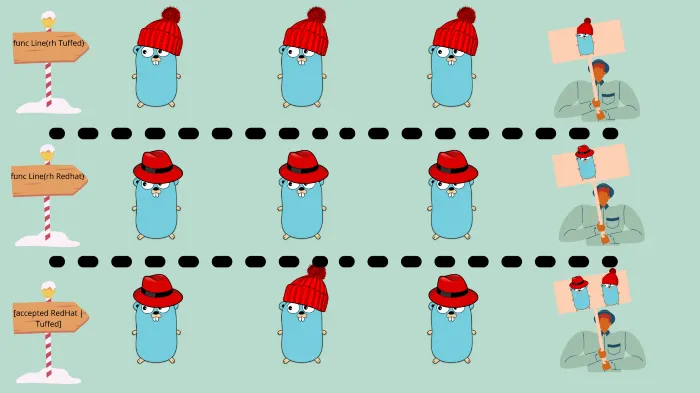A visual explanation of Generics, a line represented by a function, and the generic one accepts multiple inputs. Image by Percy Bolmér. Gopher by Takuya Ueda, Original Go Gopher by Renée French (CC BY 3.0)

Generics came to Go in update 1.18 (To be released in February 2022) with a bunch of other shiny new features. You can read about all the changes in my 1.18 patch note summary.

To get started with 1.18 you can run, or download it here.

``go install golang.org/dl/go1.18beta1@latest``

While there are other nice things added, there is no doubt that the implementation of generics has overshadowed everything else. It is a topic that has been discussed for a very long time and there are many developers for it, and many against it.

Many people find generics tricky and complex, but let’s uncover the mystery. They are not that hard to use once you get familiar with them.

In this article, we will look at what generics are and learn how to use them.

## What Are Generics And Why Does Go Need Them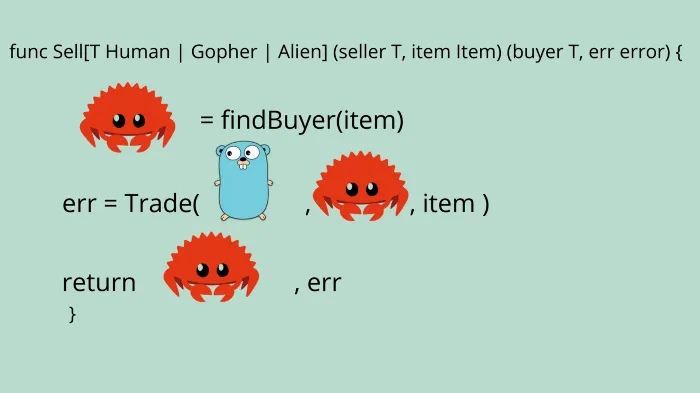Generic function, allowing multiple types of input and output. Image by Percy Bolmér. Gopher by Takuya Ueda, Original Go Gopher by Renée French (CC BY 3.0)

Generics is a way of letting a function accept multiple data types as the same input parameter. Imagine that you have a function that should accept an input parameter, and subtract the value of the second input parameter.

You would need to decide upon a certain data type to use, an int, int64, or floats. This would force any developer using the subtract function to typecast their values into the correct data type before using it.

Another solution would be to have a subtract function for int, another function for floats, etc, leaving you with multiple functions doing the same work.

What if that typecasting or duplicate functions could be skipped? In the example above, we are getting the wrong result from the float result, as we remove the 0.5 when we convert it into an int.

So, the only proper solution would be having duplicate functions, one Subtract(a,b int) int and replica Subtractfloat(a,b float32) float32.

I say the duplicate function is the only solution, I know you can use the `interface{}` input and output solution. I don’t like that hack, it is error-prone and clunky. You also lose compile-time error checks since the compiler won’t know what you are doing with that interface. This quickly becomes unmaintainable and adds a lot of extra code.

That is the issue that generics aim to solve, and why so many developers have been very eager to see it released.

The first draft of generics is released now with Go 1.18 and the solution to the above problem is simple.

## Generics And How They Work — The Basics of Generic Functions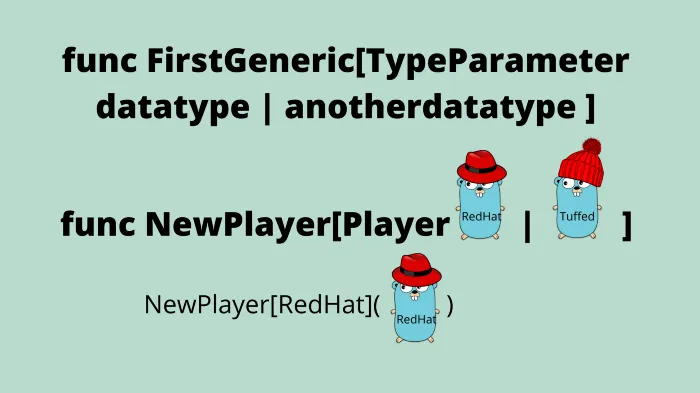Beginning to learn generics by providing Type Parameters to a first function. Image by Percy Bolmér. Gopher by Takuya Ueda, Original Go Gopher by Renée French (CC BY 3.0)

Let’s dive in and learn the basic usage of generics. We will begin with the regular Subtract function and add generic features to it while we learn what it is that we add, and why.

The syntax for functions is that we define the function by stating func FunctionName which is followed by the function parameters.

Function parameters are defined inside () and can be as many as you like. A function parameter is defined by declaring a name, followed by the data type. For instance (a int) defines that the functions local scope will have an integer named a.

The Subtract method has the function parameters a and b of data type int.

Now, function parameters might seem trivial, but it is vital to understand before traversing into generics.

Aside from function parameters, there are also Type parameters. Type parameters are defined inside [] and should be defined before the function parameters, [](a,b int). You can define a type parameter the same way you would a function parameter, the name followed by the data type.

Type parameters are usually capitalized to make them easier to spot.

An example, where we declare that the parameter V is an integer, [V int]

The difference between a function parameter and a type parameter is that the function parameter is available in the local scope of the function. If you define V the way we did above, you won’t be able to use V as a variable in the function. A type parameter only states what data types V represents.

What we define with the type parameter, is that there is a data type called V, which is an int. This allows us to use V as a replacement for int in the function parameter, and inside the scope of the function.

We can now replace the data type for a and b with V, and also the function output to V

Now, you might think that we have accomplished nothing, we simply replaced int with a more complex solution. And you are right, we are not done, the above code won’t compile. You are not allowed to replace a single data type in a type parameter as we did unless putting it in an interface.

If you try to compile the current Subtract function you should see an error stating that int is not an interface.

The reason for this is that a type parameter expects a type constraint as the value, not a data type. A constraint is an interface that the function parameters have to fulfill.

We will add a second data type to the type parameter by using a | character. The pipe character is used to say or, meaning that we can add many different data type options to the V parameter. Using the | is also a shorthand way of creating a new inline interface.

We will add int32 and float32 as possible data types to V. Doing this will automatically create an interface that is used as a type constraint by the compiler.

You can try using the new Subtract method with a simple main function.

To make things more clear, we can break the type constraint out. Just to make it a bit easier to understand, and also, allow the constraint to be reusable.

To create the constraint we simply declare an interface Subtractable with the data types to include. It is the same syntax as the shorthand definition we used in the type parameter, so we can copy that out, I also added a bunch more data types.

One last thing before you are a master of generics. You can apply a type parameter when you call the generic function to set the data type to use, this will make more sense when we get to more advanced usages.

The way to add that is to again use the type parameter, but this time in front of the function call.

``result := Subtract[int](a, b)``

## Type Arguments and Tilde (~)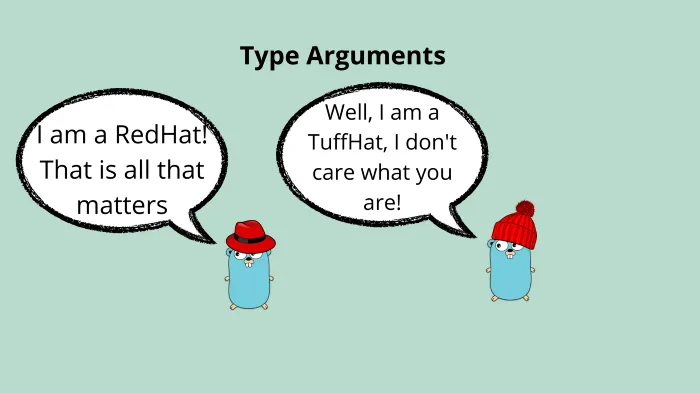Type Arguments are provided to function call to specify data type. Image by Percy Bolmér. Gopher by Takuya Ueda, Original Go Gopher by Renée French (CC BY 3.0)

So we can use generic functions by now, but there are still some details left. Imagine if you want to control the data type to use in a generic function?

Say you want to use the Subtract function that we created, it will infer the data types, unless explicitly told. You can do this by Type Arguments and the syntax is the same as when defining the generic function. You provide the data type in brackets before the parameters.

``````Subtract[int](10,20) // Will be using int datatype
Subtract[int64](10,20) // Will be using int64 datatype``````

Horray! We can now even tell the function what data type to use, excellent!

Now, there is a problem here still. What if you have a data typed that is an Alias/derived to any of the types inside Subtractable?

You won’t be able to use your data types with the way we currently declared subtractable.

``````// create a custom int derived from int
type MyOwnInteger int
var myInt MyOwnInteger
myInt = 10
Subtract(myInt, 20) // This will Crash, Since myInt is not Subtractable``````

To solve this issue, the Go team also added the ~ which tells the constraint that any type that is derived from the given type is allowed. In the example below we allow MyOwnInteger to be part of Subtractable by applying the ~ in front of it.

## Generic Types, Any & Comparable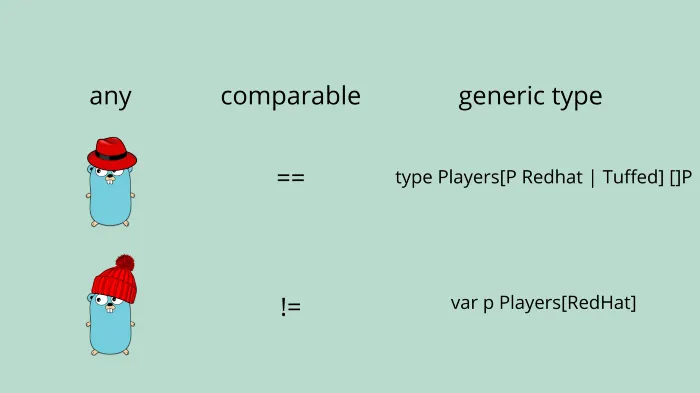New Aliases introduced in Go to be used as type constraints, any and comparable. Image by Percy Bolmér. Gopher by Takuya Ueda, Original Go Gopher by Renée French (CC BY 3.0)

We have covered generic functions and it is time to cover generic types.

To create a generic type you have to define a new type, and give it a type parameter. To learn this we will create a Results type, which is a slice of any data type found in the previously created Subtractable constraint.

The syntax should not be new, and I hope you by now can understand what is going on in the generic type definition. It is the same as in the previous examples. In the example we create a slice where the data entries will be of the data type Subtractable, thus telling the compiler that when a slice of Results is initialized, a type included in the Subtractable interface has to be defined.

To use Results we will update the main function, and notice how we have to define the data type that the Results slice is going to use when creating the variable.

Now, I already know what you are thinking, Can we say that Results should use the Subtractable type constraint?

``var resultStorage Results[Subtractable]``

Sadly, Subtractable is a type constraint, and we cant use that in the initialization of the Results. Doing so will trigger a compile error interface contains type constraints.

What we can do is use the newly introduced any to allow the Results to hold any data type. any is an alias for `interface{}`.

It is not perfect yet, it is the first draft. I hope we see a way of adding the Subtractable in the future, to avoid needing to use any since it allows us to add all kinds of data types.

There is another new type named comparable which is a type constraint that is fulfilled by any data type that can be compared by using == or !=.

It is important to get familiar with these words as you will probably see them appearing all over the as generics become more familiar to the community.

## Interface Constraints & Generic StructsInterfaces can be used as type constraints. Image by Percy Bolmér. Gopher by Takuya Ueda, Original Go Gopher by Renée French (CC BY 3.0)

So far we have only used single constraints and outputs. We have only used type constraints however it is possible to use interfaces as constraints as well.

Let us try making a generic function that accepts an interface called Moveable. The function will simply trigger the Move for the input types, any struct fulfilling this interface should be able to Move.

You should be familiar with the syntax, we create a constraint saying that the type V has to be Moveable and that the input parameter v is the type V.

We will also create a Person and a Car to try it out.

So far, we have only been using one generic parameter to keep it simple. But remember that you can add multiple, and output multiple.

Let’s combine the Moveable and Subtractable constraints to the Move function, allowing users to add a Distance value which we use to calculate how far it is until the goal.

To add more type constraints simply add parameters inside the [] just as with regular parameters. We will add the Subtractable type defined as S and instead of accepting meters as an Int, we will allow it to be S

This is how our Move function would look with both Type Constraints

``func Move[V Moveable, S Subtractable](v V, distance S, meters S) S``

However, this change alone would cause the compiler to cry because the Move function accepts an Int and the Moveable defined that this is the way Move should work. So we need to make the Move accept Subtractable.

This looks amazing right?! Sadly, the gist above is not a working example, this is just pseudo-code for what we want to accomplish. The code above will not compile, the compiler will yell at you angrily because we are using a Type Constraint inside an Interface which is not allowed, remember?

There is a way to get what we want though, Generic Structs. Generic structs are structs that get their data type defined during initialization.

Special thanks to u/ar1819 for helping me find this solution

We need to define the S type for the interface the same way we do for the generic functions. By simply adding [S Subtractable] to the interface declaration we say that not only does the Struct need the same set of methods to be part of the interface, but it also needs to be a Generic Struct.

If that is the rule for the contract, let us add that to the Car and the Person as well. Those structs will now be Generic. What this means is that when creating an object, you have to also define the data type to use for that object.

To create our Car and Person, we need to specify what data types they use. This is done as all generic features, with []. Remember, they are called Type Arguments.

Note that we are now working with Person[S Subtractable] and not only Person, so all methods also need to use this initialization.

We should also make the Move function now accept a Generic Movable by upgrading into, and also accept the Subtractable constraint.

We are ready to create the main function that uses all the things we have done. The first Move call using the Car is easy to understand, this is because we use int and the compiler will default to that.

However, the second call is more complex, since we now want to use the float64 data type. To do this we need to prepend the Move() call with the regular [] in where we define the Type Arguments. In this case, the Moveable will be a Person initialized as float64. And the datatype for Subtractable will also be a float64. So the type definition will be [Person[float64], float64]Move().

A tip is to think about the order you place the type arguments in. We can avoid the Move[Person[float64], float64] by reordering the type arguments in Move. This will work thanks to the compiler and runtime inferring datatypes.

## Conclusion

Congratulations, if you made it here you are now an expert in the subject of Generics!

I hope you will find them useful, I know many people have been waiting much for this release. Many create their libraries for Sorting slices, maps using Queues, etc. There will probably be a lot of new packages and new APIs for old libraries shortly.

Many use cases are great with generics, but sometimes it is easy to make things a bit too complex instead. Try only using them when there is an actual use case for them.

If you like my writing, don’t miss my guide for the fuzzing feature in Go, which was also released in Go 1.18!

Feel free to reach out on any of my social media below

If you enjoyed my writing, please support future articles by buying me an Coffee